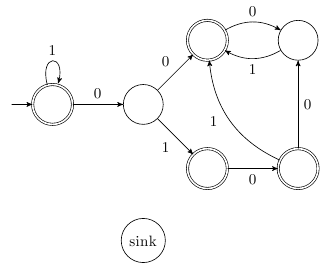# regular expressions!

#### evinda

##### Well-known member
MHB Site Helper
Hello!!!
I have to draw the DFA of the language of the following expressions:
a){$$1^*\{00,010,\varnothing\}(01)^{*}$$}
b)$$(\{\{1,0\}^{*},(\varnothing,2)^*\})^{*}$$

Could you help me to find the languages that are meant,so I can draw the DFAs?

#### Klaas van Aarsen

##### MHB Seeker
Staff member
Hello!!!
I have to draw the DFA of the language of the following expressions:
a){$$1^*\{00,010,\varnothing\}(01)^{*}$$}
b)$$(\{\{1,0\}^{*},(\varnothing,2)^*\})^{*}$$

Could you help me to find the languages that are meant,so I can draw the DFAs?
Hi evinda!!Effort?
What do you already know about DFA's and what they look like?

#### evinda

##### Well-known member
MHB Site Helper
Hi evinda!!Effort?
What do you already know about DFA's and what they look like?
I know how to draw DFAs when I have a language,but I don't know how to find the language that is represented from the expressions above.For example,if I had to draw the DFA,that accepts the language that contains the substring 001,I would do it like that:#### evinda

##### Well-known member
MHB Site Helper
How can I find,in general,the language of a regular expression??#### Evgeny.Makarov

##### Well-known member
MHB Math Scholar
How can I find,in general,the language of a regular expression??
This is just a remark (and a way to subscribe to the thread). There is no such thing as "finding the language" unless the language is finite. If the language is infinite, you cannot communicate or learn it as a set of words. Instead, you have to communicate some finite description of the language. Now, there is no canonical representation of a regular language. It can be described by a finite automaton, a regular expression, or a formula in first-order logic, and none of these is a priori better than the rest. For example, as you get familiar with regular expressions, they may become your preferred representation of regular languages.

#### evinda

##### Well-known member
MHB Site Helper
This is just a remark (and a way to subscribe to the thread). There is no such thing as "finding the language" unless the language is finite. If the language is infinite, you cannot communicate or learn it as a set of words. Instead, you have to communicate some finite description of the language. Now, there is no canonical representation of a regular language. It can be described by a finite automaton, a regular expression, or a formula in first-order logic, and none of these is a priori better than the rest. For example, as you get familiar with regular expressions, they may become your preferred representation of regular languages.
I understood...I tried to draw the finite automaton of the regular expression {$$1^*\{00,010,\varnothing\}(01)^{*}$$} and that's what I did.Is this right?Last edited:

#### evinda

##### Well-known member
MHB Site Helper
Or am I wrong???If we have this: $$\{00,010,\varnothing\}$$,do the automaton has to read all of these: $$00,010,\varnothing\$$ or just one of them?

#### Evgeny.Makarov

##### Well-known member
MHB Math Scholar
I tried to draw the finite automaton of the regular expression {$$1^*\{00,010,\varnothing\}(01)^{*}$$} and that's what I did.Is this right?
Notations for regular expressions differ. Does comma denote alternation? Is there a difference between curly braces and parentheses? Why is the complete expression surrounded by curly braces?

#### evinda

##### Well-known member
MHB Site Helper
Notations for regular expressions differ. Does comma denote alternation? Is there a difference between curly braces and parentheses? Why is the complete expression surrounded by curly braces?
Oh sorry, the complete expression is not surrounded by curly braces. It is: $$1^*\{00,010,\varnothing\}(01)^{*}$$.

With the parentheses I think that it is meant that $0$ is followed by $1$ and this sequence appears $n$ times with $n \geq 0$.

And in the curly braces the comma may denote the alternation.

#### Klaas van Aarsen

##### MHB Seeker
Staff member
Oh sorry, the complete expression is not surrounded by curly braces. It is: $$1^*\{00,010,\varnothing\}(01)^{*}$$.

With the parentheses I think that it is meant that $0$ is followed by $1$ and this sequence appears $n$ times with $n \geq 0$.

And in the curly braces the comma may denote the alternation.
Looks like your off in the right direction!!Except... that with the commas between {} that mean alternation, your graph should be slightly different...

MHB Site Helper

#### Klaas van Aarsen

##### MHB Seeker
Staff member
Looking good!One problem left. There is no such thing as an $\varepsilon$ transition.
It would be "no-input", which would not be deterministic.

Can you think of another (deterministic) transition that accepts either a '0' or a '1' that will show the same behavior?
And/or perhaps no transition at all, but marking the state as a final state?

#### Evgeny.Makarov

##### Well-known member
MHB Math Scholar
Here is my version.Edit: Made the initial state to be also accepting in order to accept 1*. Still no warranty.Thank you very much!!!